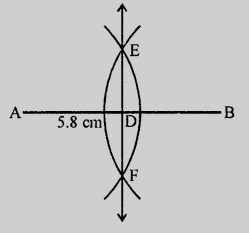# Draw a line segment $AB$ of length $5.8\ cm$. Draw the perpendicular bisector of this line segment.

Given:

Length of the line segment $AB=5.8\ cm$.

To do:

We have to draw the line segment and the perpendicular bisector of this line segment.

Solution:Steps of construction:

(i) Draw a line segment $AB = 5.8\ cm$.

(ii) With centres $A$ and $B$ and radius more than half of $AB$, draw arcs intersecting each other at $E$ and $F$.

(iii) Join $EF$ which is the required perpendicular bisector of $AB$.

Updated on: 10-Oct-2022

42 Views